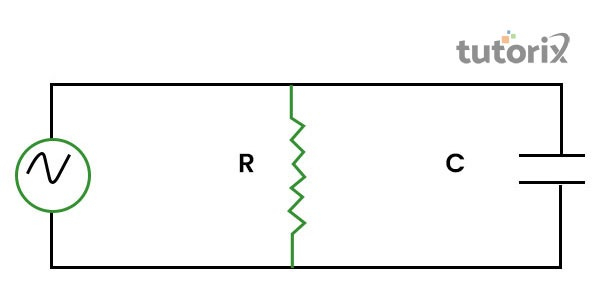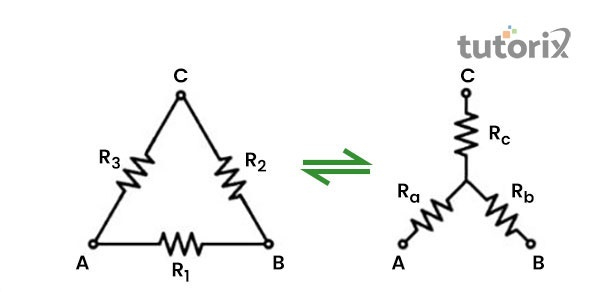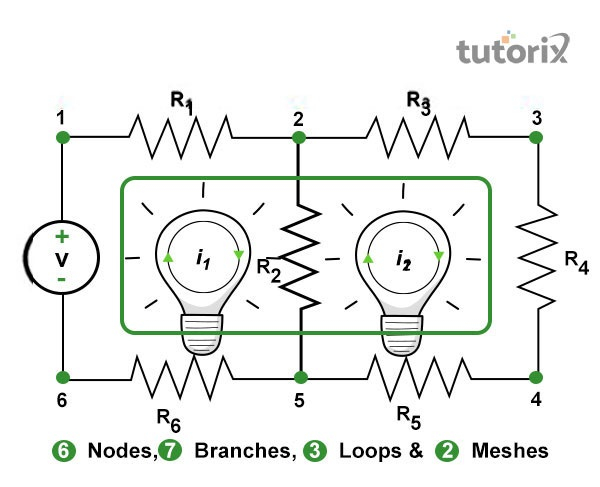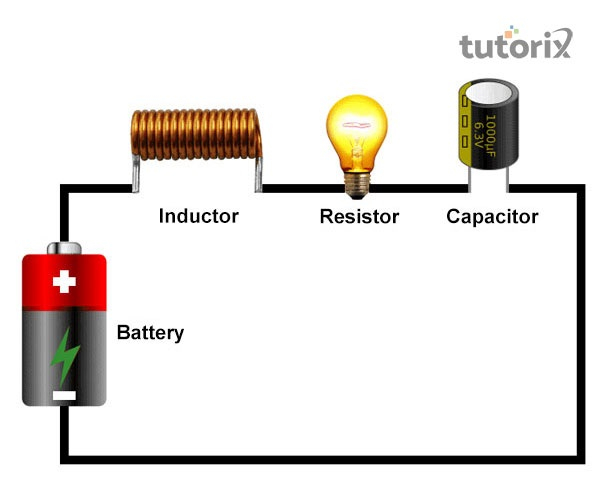# Types of Circuits

## Introduction

Energy is supplied to a circuit with a generator or a battery. In a circuit, energy is converted from different forms such as electromagnetic, chemical, thermal, and mechanical forms into electrical forms. An electrical charge is used to carry energy to the electrical circuit. Energy enters a circuit with the help of one or more than one sources. The agency of an electric charge is used in transporting electrical energy to any device.

## What is a circuit?

A circuit is a path, circular in nature with which electricity flows to a certain direction and point. In a simple circuit, three main components are available. These are the current source, load, and consulters (Thoreson & Dacey, 2019). A fixed path of electricity is also known as a circuit. In this path, a signal, data, and electricity can travel easily.

## Different types and properties of circuits

• Series circuit: In a series circuit, multiple resistances are found which are connected in a serial manner, one after another. A cascade connection is also found in this type of circuit. In this circuit, the same current is responsible for crossing each resistance.

• Parallel circuit: In this type of circuit, more than one resistance is found that is connected in a way so that each terminal is connected and forms a junction point. The other point is connected to another point.Figure 1: Parallel circuit

• Open circuit: In this circuit, no return channel is available for a continuous current flow.

• Closed-circuit: In a closed circuit, the value of current flow is determined with the help of load (Lavasani, Alavirad & Barkeshli, 2021).

• Short circuit: In a short circuit, a return channel is available to ensure a smooth current flow.

• Star-Delta circuit: In a start delta circuit, pure resistive, inductive, and capacitive circuits are included which are very different from the series, parallel circuitsFigure 2: Star delta conversion

• Linear circuit: In this circuit, constant circuit parameters are included. Some examples of such parameters are inductance, resistance, waveform, capacitance, and frequency. The parameters of this circuit do not change due to changes in voltage and current flow.

• Non-linear circuit: This circuit possesses opposite characteristics of a linear circuit. Changes in voltage and current differentiate the parameters of this circuit.

• Bi-lateral circuit: Changes in the direction of current flow do not affect the property of a bi-lateral circuit. In this circuit, the current flow in both directions.

• Unilateral unit: Function of a circuit changes due to differences in the supply of current and voltage.Rectification is not seen in the directions of current flow.

• Active circuit: One and even more than one Electro-Motive Force (EMF) is found in an active circuit.

• Passive circuit: In a passive circuit, no EMF is included.

## Circuit’s Parameters, constants, and related terms

In an electric circuit, many parts are found among which the four most important parts are node, branch, loop, and mesh.Figure 3: Nodes, branches, loops, and meshes

• Node: The junction in which more than one circuit element such as an inductor, resistor, and capacitor are connected is named a node.

• Branch: This is an individual and particular section in a circuit which is placed within two junctions (Bravyi et al. 2020). A branch helps in connecting and joining more than one part, possessing two different endpoints in a single point.

• Loops: This is a closed channel, situated within a circuit. This can also contain more than one and even more than two meshes (Antoro et al. 2020). In a loop, multiple meshes can be found. On the contrary, in a mesh, only a single loop can be situated.

• Meshes: A closed loop is a mesh that is not connected to any other loop or pathways.

## Parts of Electric Circuits and NetworksFigure 4: Parts of an electric circuit

In an electric circuit, the four most important parts are found. These are battery, inductor, resistor, and capacitor.

Battery: In a circuit, the battery is the part that helps in storing chemical energy. The chemical energy is converted into electrical energy with the help of a battery.

Inductor: In an inductor, energy is stored in form of magnetic energy during the time of energy flow.

Resistor: This is a two-terminal electrical component that is used in implementing electrical resistance (Rosdianto, 2019). This helps in reducing current flow, dividing voltages, and adjusting signal levels.

Capacitor: This part of a circuit helps in storing electrostatic energy.

## Conclusion

A significant conductive path for flowing electricity or current is known as an electric circuit. The relation between the source of load and voltage is established by using a conductive wire. In between the load and the source of voltage, a fuse and on / off switch is available. Based on different types of flow in current, circuits can be divided into two parts, namely DC and AC circuits. Other types of circuits such as parallel, series, closed, short, and open circuits are also important circuits, used in the current transmission process.

## FAQs

Q1. What are the five most important types of an electric circuit, available for energy transmission?

Ans. The five most important circuits are open circuit, close circuit, parallel circuit, series circuit, and short circuit. Active circuits and passive circuits are also two other most important types of circuits that help in the energy transmission process.

Q2. What are the two basic electric circuits?

Ans. Two of the most important and basic types of circuits are regarded as series and parallel circuits. Other circuits are also available there that helps in transmitting energy in a device.

Q3. How a series circuit is different from a parallel circuit?

Ans. In a series circuit, a single pathway is seen. In a parallel circuit, multiple current pathways are found.

Q4. What is a closed circuit?

Ans. In a closed circuit, good electrical connectivity is available in which no interruption is recorded. The current, flowing within a closed circuit faces a smooth continuity during current flow.